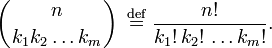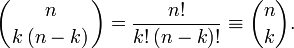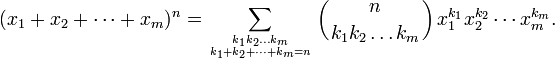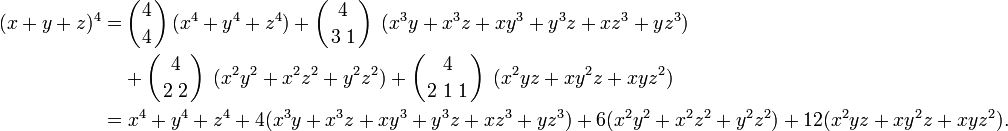# Multinomial coefficient

In discrete mathematics, the multinomial coefficient arises as a generalization of the binomial coefficient.

Let k1, k2, ..., km be natural numbers giving a partition of n:$k_1 + k_2 + \cdots + k_m = n.$

The multinomial coefficient is defined by$\left({n \atop k_1 k_2\ldots k_m}\right)\;\stackrel{\mathrm{def}}{=} \; \frac{n!}{k_1!\,k_2!\,\ldots k_m!} .$

For m = 2 we may write:$k_1 \equiv k \quad\hbox{and}\quad k_2 = n-k,$

so that$\left({n \atop k\, (n-k)}\right)= \frac{n!}{k!\,(n-k)!} \equiv \binom{n}{k}.$

It follows that the multinomial coefficient is equal to the binomial coefficient for the partition of n into two integer numbers. However, the two coefficients (binomial and multinomial) are notated somewhat differently for m = 2.

The multinomial coefficients arise in the multinomial expansion$(x_1+x_2+ \cdots+x_m)^{n} = \sum_{k_1 k_2\ldots k_m \atop k_1+k_2+\cdots+k_m=n} \left({n \atop k_1 k_2\ldots k_m}\right) x_1^{k_1}x_2^{k_2} \cdots x_m^{k_m}.$

The number of terms in this expansion is equal to the binomial coefficient:$\binom{n+m-1}{n}$

Example.   Expand (x + y + z)4:$m=3,\;n=4\;\quad\Longrightarrow\quad \binom{n+m-1}{n} = \binom{6}{4} = 15$

The 15 terms are the following:\begin{align} (x+y+z)^4 = & \left({4 \atop 4}\right)(x^4 + y^4 + z^4) + \left({4 \atop 3\; 1}\right)\; (x^3y + x^3z + xy^3 + y^3z + xz^3 + yz^3) \\ &+ \left({4 \atop 2\; 2}\right)\; (x^2y^2 + x^2z^2 + y^2z^2) + \left({4 \atop 2\; 1\;1}\right)\; (x^2 y z + xy^2z + xy z^2) \\ =& \;x^4 + y^4 + z^4 + 4(x^3y + x^3z + xy^3 + y^3z + xz^3 + yz^3) + 6(x^2y^2 + x^2z^2 + y^2z^2) + 12(x^2 y z + xy^2z + xy z^2). \end{align}

A multinomial coefficient can be expressed in terms of binomial coefficients:$\left({k_1+k_2+\cdots+k_m \atop k_1 k_2\ldots k_m}\right) = \binom{k_1+k_2}{k_1}\binom{k_1+k_2+k_3}{k_1+k_2}\cdots\binom{k_1+k_2+\cdots+k_m}{k_1+\cdots+k_{m-1}}$

## Reference

D. E. Knuth, The Art of Computer Programming, Vol I. Addison-Wesley, Reading Mass (1968) p. 64